• 基于DSP代码自动生成技术实现FIR低通滤波器、电子技术,开发板制作交流Matlab DSP
• 利用matlab代码来实现fdatool生成滤波器系数的功能（FIR 低通滤波器） 用matlab设计滤波器时，我们会使用到fdatool工具， 首先大概说一下这个工具的使用。 假设我们需要设计一个滤波器： 采样率Fs为30.72MHz。 通带...

提示：文章写完后，目录可以自动生成，如何生成可参考右边的帮助文档

利用matlab代码来实现fdatool生成滤波器系数的功能（FIR 低通滤波器）

用matlab设计滤波器时，我们会使用到fdatool工具，

首先大概说一下这个工具的使用。

假设我们需要设计一个滤波器：
采样率Fs为30.72MHz。
通带Fpass: 9 MHz,
阻带Fstop: 10MHz
带内波动: Apass: 0.1
带外抑制: Apass: 80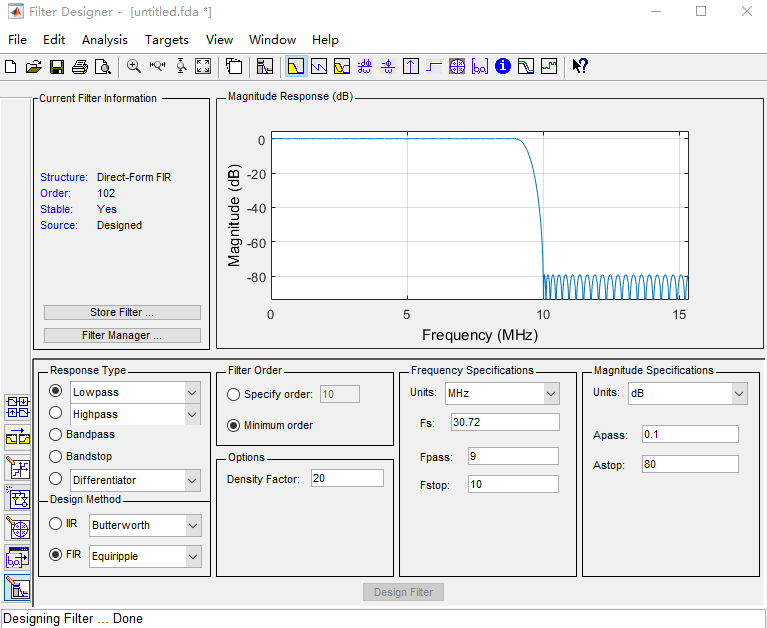定点化，将系数归一化，设置如下图，确保输入通带的波形和输出波形幅值基本不变。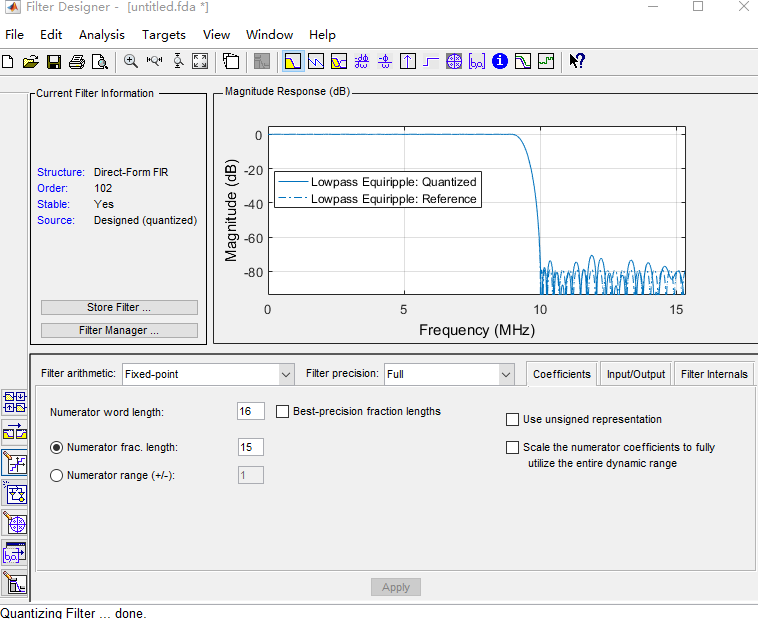生成的COE文件可以直接被XILINX的 IP核使用。

如果上述生成滤波器系数的步骤，可以通过matlab代码来实现，则会更加的事半功倍。
例如，假如有一个这样的需求
采样率Fs为80MHz。
通带Fpass: 9+n0.5 MHz, (0<=n<=40)
阻带Fstop: 10+n
0.5MHz (0<=n<=40)
带内波动: Apass: 0.1
带外抑制: Apass: 80
需要生成41组COE，每次都通过fdatool工具来进行生成，那将是繁琐的。而我们可以通过以下代码来简化工作：

figure(1);

Apass = 0.1;
Astop = 80;
Fs = 30.72;
Fpass = 9;
Fstop = 10;

dev = [(10^(Apass /20)-1)/(10^(Apass /20)+1)  10^(-Astop/20)];
[n,fo,ao,w] = firpmord([Fpass Fstop] ,[1 0],dev,Fs);
b = firpm(n,fo,ao,w,{20});
bb = round(b*2^15);
plot(bb);
grid;
hold on;
I = find(bb < 0); bb(I) = 65536 + bb(I);
c = [ dec2hex(bb)];
fid1 = fopen('d:\filter1.coe','w');
fprintf(fid1,';Create by gzy de tool \n');
fprintf(fid1,'Coefficient_Width = 16;\n');
fprintf(fid1,'CoefData = \n');
for i = 1 : length(b)-1
fprintf(fid1,'%s,\n',c(i,end-3:end));
end;
fprintf(fid1,'%s;\n',c(end,end-3:end));
fclose(fid1);

上面的代码与fdatool生成的定点化系数完全一致。

写于2021年10月11日。
如需交流，QQ：172146579

展开全文matlab
• 本文使用的开发环境为：Win10 Matlab... 按照下图调整FIR低通滤波器的参数：我们设计的是采样频率100Hz，截止频率10Hz的8阶FIR低通数字滤波器。 生成matlab函数代码：File—Generate MATLAB Code—Filter Design F...

本文使用的开发环境为：Win10 Matlab2018a 版本。

在matlab命令窗口输入：filterDesigner命令，即可打开filterDesigner设计工具。按照下图调整FIR低通滤波器的参数：

我们设计的是采样频率100Hz，截止频率10Hz的8阶FIR低通数字滤波器。生成matlab函数代码：File—Generate MATLAB Code—Filter Design Function

我在这里将生成的m文件保存为fir_8.m。在matlab中打开fir_8.m是这个样子的：

代码如下：function Hd = fir_8

%FIR_8 Returns a discrete-time filter object.

% MATLAB Code

% Generated by MATLAB(R) 9.4 and DSP System Toolbox 9.6.

% Generated on: 09-Apr-2019 11:22:46

% FIR Window Lowpass filter designed using the FIR1 function.

% All frequency values are in Hz.

Fs = 100; % Sampling Frequency

N = 7; % Order

Fc = 10; % Cutoff Frequency

flag = 'scale'; % Sampling Flag

% Create the window vector for the design algorithm.

win = hamming(N+1);

% Calculate the coefficients using the FIR1 function.

b = fir1(N, Fc/(Fs/2), 'low', win, flag);

Hd = dfilt.dffir(b);

% [EOF]

接下来我们要设计一个带噪声的信号函数，然后通过这个滤波器，来测试滤波效果：

在matlab中新建一个脚本，保存为signal.m，与fir_8.m保存在同一文件夹中。

代码如下：x = 0:0.01:4;%定义400个取样点

y = 0.1*sin(pi*x) +0.1*sin(2*pi*49*x);%设计含有高频信号与低频信号的输入信号

figure(1);

plot(x,y);%画出输入信号图形

title('输入信号');

Hd = fir_8;%引入滤波器,Hd包含了fir_8滤波器的各项参数

d = filter(Hd,y);%通过filter函数将信号y送入参数为Hd的滤波器，输出信号d

figure(2);

plot(x,d);%画出通过滤波器的信号d的波形

title('输出信号');

figure(3);

plot(x,y,'r');%画出输入信号图形

hold on;%保持画出的输入信号图形

plot(x,d,'b');%画出输出信号波形

title('输入/输出信号');

legend('输出信号','输入信号');

运行signal.m(在matlab命令行窗口输入signal即可)PS: 本文主要参考惠飞《FPGA嵌入式系统开发与实例》第5.4.3节

代码文件下载

展开全文• matlab数字低通滤波器 MATLAB一阶低通滤波器的设计截止频率50Hz，设计MATLAB低通滤波器的程序及注释，谢谢 %滤波前数据t = 0:0.01:10;f=5*sin(2*pi*20*t)+5*sin(2*pi*60*t)；%滤波器设计Fpass = 1; % Passband ...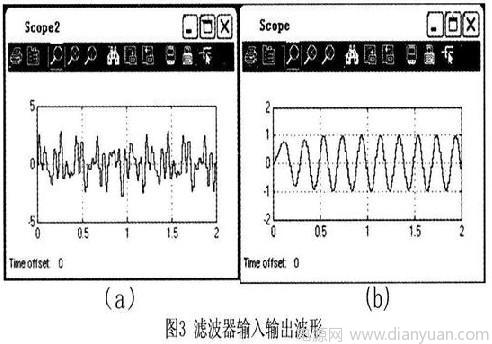matlab数字低通滤波器 MATLAB一阶低通滤波器的设计

截止频率50Hz，设计MATLAB低通滤波器的程序及注释，谢谢 %滤波前数据t = 0:0.01:10;f=5*sin(2*pi*20*t)+5*sin(2*pi*60*t)；%滤波器设计Fpass = 1; % Passband FrequencyFstop = 50; % Stopband FrequencyApass = 1;% Passband Ripple (dB)Astop = 60, Fpass;.lowpass(';fp;):0,ast$matlab数字低通滤波器。', , Astop;equiripple'.01., '. ',f2, Apass;StopbandShape',fst, ;b'),ap,'%滤波前数据t = 0。 擅长：VC++ 其他编程语言 工程技术科学 环境学根据你的题目设计一个butterworth的3阶滤波器 N=3; [z,p,k]=buttap(N)；求出该滤波器的零点和极点 OmegaC=2*pi*50； 给定的截止频率 p=p*OmegaC； 由于前面是归一化的，现在还原 k=k*OmegaC^N; >> k=k*OmegaC^$matlab数字低通滤波器。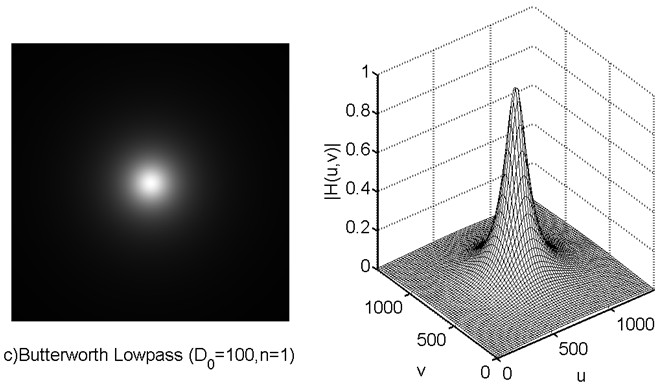用matlab设计低通滤波器。IIR butterworth的，fdatool产生的：function Hd = test1%TEST1 Returns a discrete-time filter object.%% M-File generated by MATLAB(R) 7.0.4 and the Signal Processing Toolbox 6.3.%% Generated on: 25-Jun-2009 15$matlab数字低通滤波器。 帮忙编一个matlab的低通滤波器的程序。p2)I=[]；%绘制数字滤波器的幅频特性和相频特性[db,a]=zp2tf(z; A(fix((i+1)/2); ).0001)&nbsp,'&nbsp.5*cos(2*pi*f2*t),Arow]=residuez(Brow; &nbsp:)=real(Brow); for ):),1；%输入信号subplot(2;);; &nbsp,a;&n$matlab数字低通滤波器。xlabel('%频带变换得到归一化滤波器[N; 输出信号',;s'endendI=I' %用设计好的滤波器对信号进滤波处理figure(4)f1=500;&nbsp,i]; xlabel(' &nbsp,a1; &nbsp,;&nbsp,; = s'filter'&nbsp,title('&nbsp,a)[H;&nbsp:i+1,w1),Arow; &nbsp,az); [Brow; &nbsp:)=real(Brow); for &nbsp,1000-0000*eps); ylabel(' B(fix((i+1)/2); &nbsp,y1,x);&nbsp,-30,A1]=dir2par(bz; [Brow; Arow=p(i;&nbsp:N-1; &nbsp,w0]=freqs(b,Arow]=residuez(r(N-1); $matlab数字低通滤波器。 matlab 数字带通滤波器。想设计一个带通滤波器，比如通带范围为100~200Hz？谢谢求各位高人帮忙。 怎么用matlab实现？谢谢，帮助。 以前我做的一个完整的信号滤波前后的程序，可能数据有些不一样，但用MATLAB做滤波器的大体思路都差不多，希望对你有用。(完全自创，要给分哦)f=8*10^3； %信号频率f1=30*10^3；%噪声频率l=100; %信号的长度fs=100*10^3; %采样频率%%%%%%%%%%%%%%%%生成信号t=(0:l-1)/fs;s=0.5*sin(2*pi*f*t)+cos(2*pi*f1*t);figure(1);subplot(1,2,1);plot(fs*t(1:60),s(1:60)); title('时域波形');xlabel('时间');ylabel('幅度$matlab数字低通滤波器。k=fs*linspace(0,1,nf)-fs/2;subplot(1,2,2);plot(k,abs(Y)); %信号的频谱图title('信号频谱');xlabel('频率');ylabel('幅度');grid on;%%%%%%%%%%%%%%%%%%%%%%%%%%%%%%滤波器设定ws=10*10^3; %通带截止频率ws1=20*10^3; %阻带起始频率ds=50; $matlab数字低通滤波器。 Matlab的FIR低通滤波器阶数取多少为好。这要看你过渡带的归一化长度，还有阻带的衰减情况(或者通带波纹，阻带波纹)。你可以用matlab的freqz函数看一下FIR滤波器系数的频率响应情况怎样。这个没有说取多少阶为好。你可以用matlab的freqz函数看一下FIR滤波器系数的频率响应情况怎样，根据实际需要把这要看你过渡带的归一化长度，还有阻带的衰减情况(或者通带波纹。 Matlab的FIR。 基于MATLAB的FIR低通滤波器程序。你目前给出的技术指标不完全，还需要采样频率、通带波纹、阻带衰减。给全了倒是可以帮你编一个。那就假设几个吧：采样频率：Fs=80KHz经计算得相应数字滤波器的指标：通带截止频率：fp=0.6*Fs/2通带波纹：rp=0.01(假设的，单位dB，下同)；阻带截止频率：fs=0.7*Fs/2阻带衰减：rs=0.1(假设的，同上)程序如下，给出了幅频特性曲线。clear;Fs=80;fp=0.6*Fs/2$matlab数字低通滤波器。

试调用MATLAB函数设计数字低通滤波器,高通滤波器,,,,。。1.试调用MATLAB函数设计数字低通滤波器，截止频率wc=0.2pi,过渡带宽度△w<0.4pi,阻带衰减As>40dB.

2.试调用MATLAB函数设计数字高通滤波器，要求通带截止频率wp=0.5pi,阻带截止频率ws=0.25pi，通带最大阻带衰减Ap=1dB，阻带最小阻带衰减As=40dB.

3.试调用。。 调用MATLAB函数设计数字低通滤波器包哦。 你好，帮在你设计好啊。

求 MATLAB 巴特沃思 低通滤波器程序。冲击响应不变法函数 [bz,az]=impinvar(b,a,Fs) [bz,az]=impinvar(b,a) 例如：取采样频率f=1KHz，用双线性变换法设计五阶Butterworth低通数字滤波器，绘出模拟滤波器与数字滤波器的幅频与相频特性，MATLAB程序如下： [z,p,k]=buttap(5) ；% 设计五阶Butterworth低通模拟滤波器原型 [zd,pd,kd]=bilinea$matlab数字低通滤波器。w=128; freqs(b,a,w) figure; freqz(b,a,w) 实例：1、设带通滤波器的滤波器中心频率为W0=2KHz,带宽为BW=100Hz, 取采样频率f=10kHZ,用脉冲相应不变法设计，设计五阶带通Butterworth数字滤波器,绘出数字滤波器的频谱特性 [z,p,k]=buttap(5); [b,a]=zp2tf(z,p,k); w=128; w0=2000; [bt,at]=lp2bp(b,a,w0,10000); [bz,az]=impinvar(b,a,w); freqz(bt,at,w) 2、直接设计五阶butterworth带通滤波器，绘出频谱图。(高端与低端截止频率分别为0.2和0.9) figure; w=[0.2,0.9]; [b,a]=butter(5,w);$matlab数字低通滤波器。

matlab使用低通滤波器来处理数据。先设计数字滤波器 fir2(N,f,m) 其中N是滤波器长度 f是截止频率(归一化为0到1) m是该截止频率对应的滤波器相应 我说的比较简单 去查一下help 讲的很详细当然还有其他方法设计数字滤波器 根据你的需要选择吧得到滤波器后 与10000个点做卷积就可以了 最后还可以用freqz命令做出滤波结果的频率响应希望能帮到你。

用MATLAB设计低通数字滤波器的参数计算问题！利用MATLAB的FDATOOL设计请帮我计算出 用什么窗函数，Beta值，Specify order，并附详细计算方法。 一般用的都是butterworth的，以保证通带内平坦，阶数的计算方法公式编辑器编辑的公式复制不了，matlab语言了，n=log10(10.^(0.1*As-1))/log10(ws)；%最小阶数，ws是止带归一化频率，As是止带衰减，没必要自己算设置minimum,order就可以。

数字滤波器由数字乘法器、加法器和延时单元组成的一种算法或装置。数字滤波器的功能是对输入离散信号的数字代码\$matlab数字低通滤波器。对于低通滤波器，一般用的都是butterworth的，以保证通带内平坦，阶数的计算方法公式编辑器编辑的公式复制不了，matlab语言了

n=log10(10.^(0.1*As-1))/log10(ws)；%最小阶数

ws是止带归一化频率

As是止带衰减

其实没必要自己算设置minimum order就行了。

展开全文• FDATool设计FIR低通滤波器

千次阅读 2018-12-26 12:40:43
（一） 设计一个低通滤波器  采样频率 Fs = 50Hz Fpass = 10Hz Fstop = 20Hz 打开matlab，键入fdatool后，会弹出该工具的对话框。然后按照下图设置相关参数，参数列表中的Fs应该是采样频率，Fc是截止频率，即...

（一） 设计一个低通滤波器

采样频率 Fs = 50Hz   Fpass = 10Hz   Fstop = 20Hz

打开matlab，键入fdatool后，会弹出该工具的对话框。然后按照下图设置相关参数，参数列表中的Fs应该是采样频率，Fc是截止频率，即幅频特性曲线-3dB对应的频率。点击“Design Filter”后，幅频特性曲线会被更新。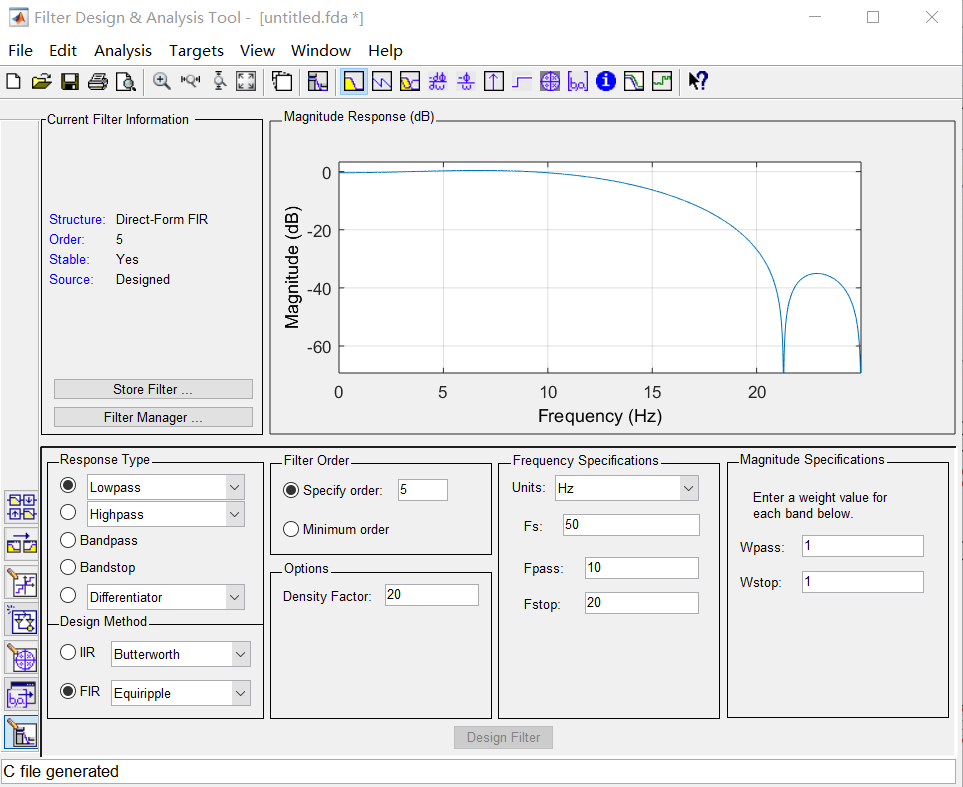Targets -> generate C Header  生成的头文件

/*
* Filter Coefficients (C Source) generated by the Filter Design and Analysis Tool
* Generated by MATLAB(R) 9.0 and the Signal Processing Toolbox 7.2.
* Generated on: 26-Dec-2018 09:54:34
*/

/*
* Discrete-Time FIR Filter (real)
* -------------------------------
* Filter Structure  : Direct-Form FIR
* Filter Length     : 6
* Stable            : Yes
* Linear Phase      : Yes (Type 2)
*/

/* General type conversion for MATLAB generated C-code  */
#include "tmwtypes.h"
/*
* Expected path to tmwtypes.h
* C:\Program Files\MATLAB\R2016a\extern\include\tmwtypes.h
*/
const int BL = 6;
const real64_T B = {
-0.08631845386171,   0.0581511407323,   0.5053647362323,   0.5053647362323,
0.0581511407323, -0.08631845386171
};   //Fir 滤波器参数

（二 ）Matlab 脚本测试

clear all;

Ts = 0.02;      %采样时间
%FDAtool设计的参数  Target -> generate C header
b = [ -0.08631845386171    0.0581511407323    0.5053647362323    0.5053647362323  0.0581511407323  -0.08631845386171];  %滤波器参数
ss = [0 0 0 0 0 0];
for i = 1:1:100
time(i) = i * Ts;
x(i) = 2*sin(10 * pi * time(i)) + cos(40 * pi * time(i)) + 0.1 * rands(1);        %5Hz 20Hz 原信号

%代数方法求滤波后的波形
ss(6) = ss(5);
ss(5) = ss(4);
ss(4) = ss(3);
ss(3) = ss(2);
ss(2) = ss(1);
ss(1) = x(i);
zz(i) = b(1) * ss(1) +  b(2) * ss(2) +  b(3) * ss(3) +  b(4) * ss(4) +  b(5) * ss(5) +  b(6) * ss(6);
end

yy = filter(b,1,x);         %使用 filter 函数执行滤波器
figure(1);
plot(time,x,'b',time,yy,'r',time,zz,'y+');
xlabel('秒');

%% 傅里叶变换，左半,检查滤波效果
figure(2)
fy = fft(x);
fyy = fft(yy);
fzz = fft(zz);
i=1:1:100;
plot(i,abs(fy),'y',i,abs(fyy),'g+',i,abs(fzz),'r-');
xlabel('1/2 Hz');
ylabel('幅度乘以采样频率')

（三）仿真结果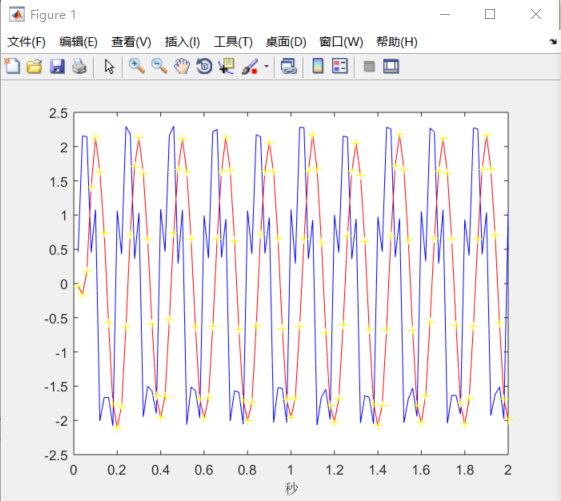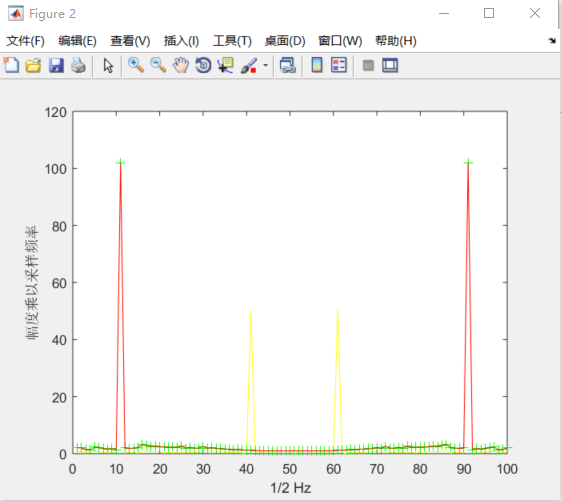20Hz的信号被过滤掉了

展开全文•   本文我们学习低通滤波器的设计。 1、问题描述   现在考虑我们有信号m(t)=Rect(t−τ/2τ)m(t)={\rm Rect}(\frac{t-{\tau}/{2}}{\tau})m(t)=Rect(τt−τ/2​)为矩形脉冲，波形如下 m(t)m(t)m(t)的傅立叶变换...
• Matlab设计和实现IIR低通滤波器对于智能车的车友来说，滤波器在处理比赛任务相当有效，像这样处理传感器数据q = 0.95;y = y*q + x*(1-q);如果需要更高性能的滤波器怎么办？matlab提供了IIR 滤波器设计设计工具，IIR ...
•matlab 开发语言
• 本程序是窗函数法设计FIR数字滤波器Matlab程序——矩形窗
• Matlab生成fir滤波器抽头系数

万次阅读 多人点赞 2018-12-20 20:09:35
1、 打开 MATLAB 软件，在命令窗口输入 fdatool 并回车，就会弹出滤波器设计工具 2、 FIR滤波器设计方法有多种，，最常用的是窗函数设计法（Window）、等波纹设计法（Equiripple）和最小二乘法 （Least-Squares）...fir滤波器 matlab tap xilinx
• 一、摘要前面一篇文章介绍了通过FDATool工具箱实现滤波器的设计，见“二、实验平台Matlab7.1三、实验原理以低通滤波器为例，其常用的设计指标有：通带边缘频率fp(数字频率为Ωp)阻带边缘频率fst(数字频率为Ωst)通带...
• fir滤波器matlab程序，包括低通、高通、带阻、带通，注释非常详尽，大家可以对里面的相关参数进行修改，比如截止频率，窗口类型等，而且对滤波前后波形进行了频谱分析，以验证滤波的效果，非常直观，很有利于初学者...
• Fir低通滤波器（Verilog手写代码）

千次阅读 多人点赞 2020-03-19 01:00:51
Fir低通滤波器（Verilog手写代码） 对于fir低通滤波器，现在很多视频上讲的都是调用IP核，的确，firIP核既简单又快捷，而且效果还不错，但是在使用上也会受限，毕竟他的抽头系数是先固定好的。现在小编来教大家用...verilog 经验分享 matlab
• 本文档为纯代码。在MATLAB中，设计IIR和FIR滤波器分别对一定频率的音频信号进行滤波，滤除高频噪声，程序中所加载的噪声是利用正弦函数生成的高频噪声，滤波器为低通滤波器
• 使用MATLAB生成滤波器有很多学问，这里只是作为初步的探索，和FPGA的更多结合，也正在探索中，相关博文例如：【 FPGA 】FIR滤波器目录，该专题目录正在记录我学习FIR滤波器的过程。 MATLAB生成30阶低通1MHz海明窗...
• 用MATLAB设计FIR滤波器

千次阅读 多人点赞 2021-01-28 13:10:07
MATLAB设计FIR滤波器滤波器滤波器定义滤波器种类滤波器设计滤波器设计要求Matlab程序设计滤波器利用Matlab工具箱设计滤波器总结 滤波器 滤波器定义 “滤波器(filter),是一种用来消除干扰杂讯的器件,将输入或输出...matlab 数字信号处理
• Matlab代码verilog FIR_Filters SV和Verilog中的低通滤波器 SV文件包含一个完全可自定义的FIR滤波器，可以在其中更改抽头的数量，并且可以...Verilog文件包含两个硬编码的FIR低通滤波器，其值由matlab中的FDA工具确定。
• 为保证所设计的FIR数字低通滤波器具有严格的线性相位,在对几种FIR基本结构的比较之后,采用了线性相位FIR滤波器的直接型结构。使用Matlab内置函数计算出滤波器的系数和检验滤波器的频率响应特性。采用C语言实现数字...
• DSP设计滤波器报告姓名：张胜男 班级：07级电信(1)班 学号：一低通滤波器的设计(一)实验目的：掌握IIR数字低通滤波器的设计方法。(二)实验原理：1、滤波器的分类滤波器分两大类：经典滤波器和现代滤波器。经典滤波器...
• 设定低通滤波器的采样频率为112MHz，信号带宽为30MHz,，那么对于同相与正交分量分别具有15MHz带宽，因此低通滤波器的通频带为15MHz，参数配置如下(在MATLAB中调用fdatool函数) ...verilog fpga 数字信号处理
• 红色框设置滤波器类型，（这里设置为发送与接收滤波中常用的升余弦FIR滤波器） ； 橙色框设置滤波器阶数，此处设置为64阶，产生65个系数； 蓝色框设置窗函数类型，这里选择哈明窗； 绿色框设置带宽与单边带截止频率...滤波器 Matlab
• 第37章 STM32F429的FIR低通滤波器实现（支持逐个数据的实时滤波） 本章节讲解FIR低通滤波器实现。 目录 37.1 初学者重要提示 37.2 低通滤波器介绍 37.3 FIR滤波器介绍 37.4 Matlab工具箱filterDesinger生成低...
• MACROBUTTON MTEditEquationSection2 SEQ MTEqn \r \h \* MERGEFORMAT SEQ MTSec \r 1 \h \* MERGEFORMAT SEQ MTChap \r 1 \h \* MERGEFORMAT汉宁窗设计线性相位FIR低通数字滤波器学校 宜 宾 学 院院系 物理与电子......

matlab生成fir低通滤波器matlab 订阅